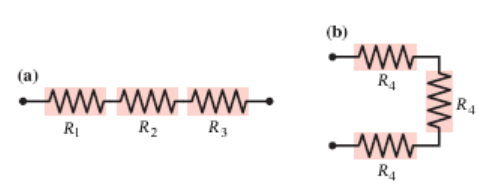# Problem: What is the equivalent resistance of each group of resistors shown in the figure below? In the figure, R1 = 2.0 ?, R2 = 3.5?, R3 = 7.5 ?, and R4 = 2.0 ?.(a) ______ ?(b) ______ ?

###### FREE Expert Solution

Equivalent resistance for series capacitors:

$\overline{){{\mathbf{R}}}_{\mathbf{e}\mathbf{q}}{\mathbf{=}}{{\mathbf{R}}}_{{\mathbf{1}}}{\mathbf{+}}{{\mathbf{R}}}_{{\mathbf{2}}}{\mathbf{+}}{{\mathbf{R}}}_{{\mathbf{3}}}}$

(a)

The resistors are in series:###### Problem Details

What is the equivalent resistance of each group of resistors shown in the figure below? In the figure, R1 = 2.0 ?, R2 = 3.5?, R3 = 7.5 ?, and R4 = 2.0 ?.

(a) ______ ?

(b) ______ ?